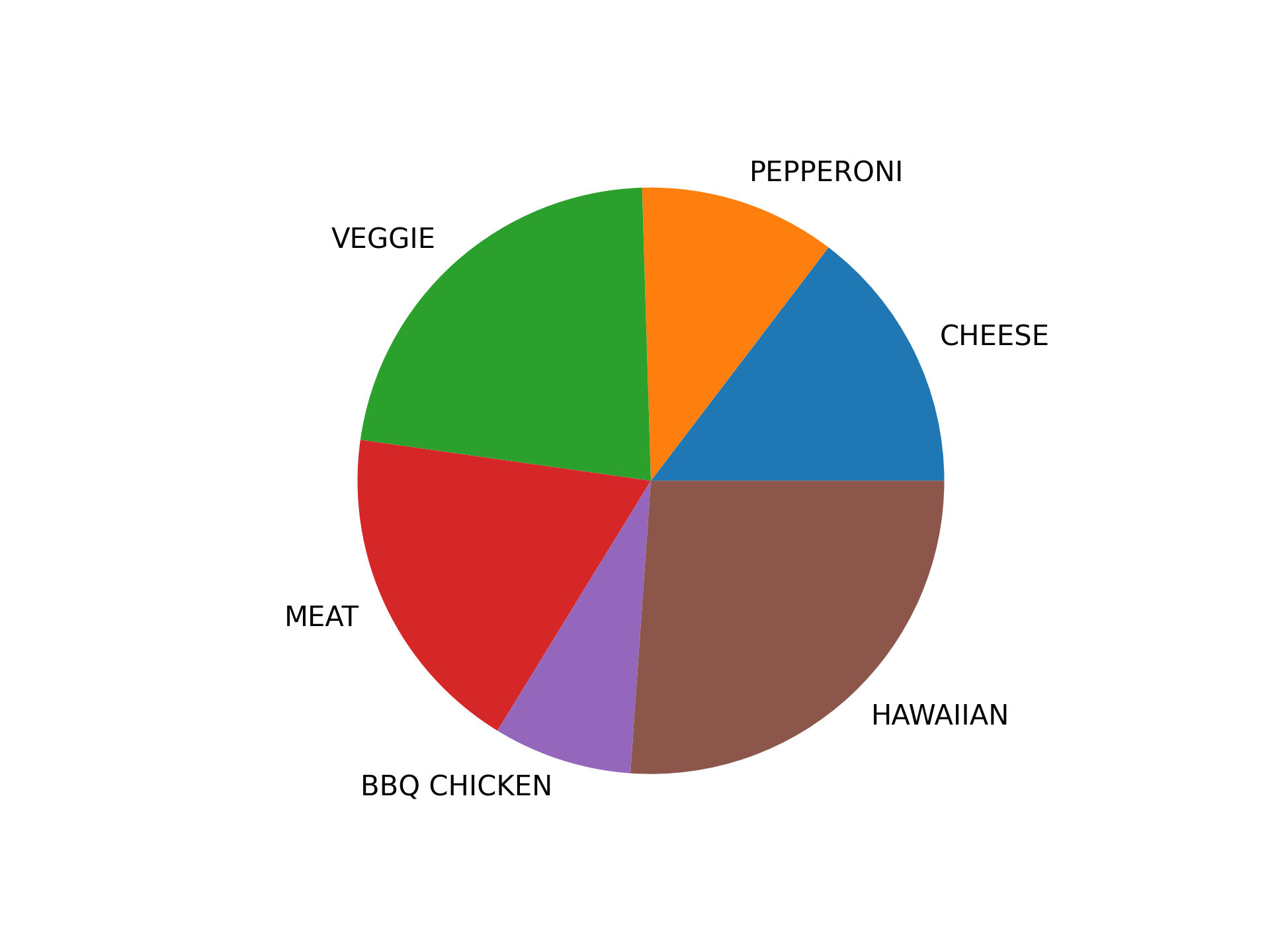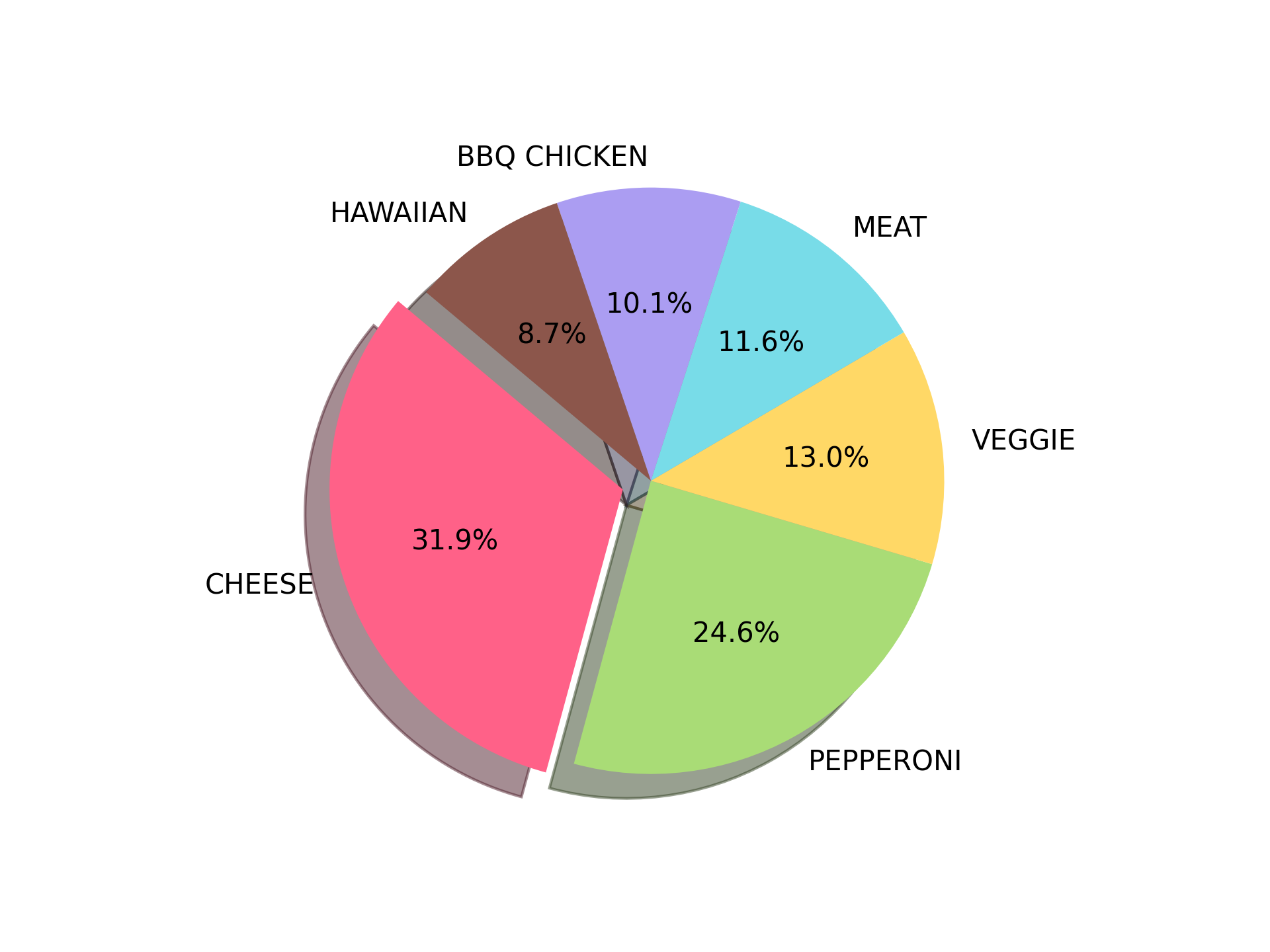# .pie()

The `.pie()` method in the Matplotlib library is used to draw a pie chart, a common format that shows the percentage of individual elements with respect to the data as a whole.

## Syntax

``````matplotlib.pyplot.pie(x, explode, labels, colors, autopct, pctdistance, shadow, labeldistance, startangle, radius, counterclock, wedgeprops, textprops, center, frame, rotatelabels, normalize, hatch, data)
``````

The `x` parameter, the data to be mapped, is required and represents a 1D array-like object. Other parameters are optional and modify plot features like wedge colors and labels.

`.pie()` takes the following arguments:

• `x`: 1D array-like object that specifies the wedge sizes.
• `explode`: Array-like object which specifies the fraction of the radius with which to offset each wedge.
• `labels`: A list of strings to provide labels for each wedge.
• `colors`: Array-like sequence of colors that the pie chart will cycle through.
• `autopct`: A string or function used to generate labels inside the wedges showing their numeric value.
• `pctdistance`: The relative distance along the radius to draw the text generated by `autopct`.
• `shadow`: Boolean value indicates if a shadow is drawn beneath the pie.
• `labeldistance`: The relative distance along the radius to draw the labels.
• `startangle`: An angle, counterclockwise from the x-axis, at which to rotate the start of the pie.
• `radius`: The radius of the pie.
• `counterclock`: Boolean to specify clockwise or counterclockwise direction for fractions.
• `wedgeprops`: Dict of arguments to pass to each `patches.Wedge`.
• `textprops`: Dict of arguments passed to the text objects.
• `center`: (float, float), the center coordinates of the chart.
• `frame`: Bool, determines if Axes frame is plotted with the chart.
• `rotatelabels`: If `True`, rotates each label to the angle of the corresponding slice.
• `normalize`: Bool, when `True`, always make a full pie by normalizing x, otherwise results in a partial pie.
• `hatch`: Str or list, hatching pattern applied to all pie wedges or sequence of patterns that the chart will cycle through.

## Examples

Examples below demonstrate the use of `.pie()` to plot values and show the functionality of some arguments.

A simple pie chart:

```# Import librariesfrom matplotlib import pyplot as plt
# Creating datasetpizza = ['CHEESE','PEPPERONI','VEGGIE','MEAT','BBQ CHICKEN','HAWAIIAN']data = [23,  17,  35,  29,  12,  41]
# Creating plotplt.pie(data, labels = pizza)
# show plotplt.show()
```

Output:Customizing the pie chart:

```# Import librariesimport matplotlib.pyplot as plt
# Plot datapizza = ['CHEESE','PEPPERONI','VEGGIE','MEAT','BBQ CHICKEN','HAWAIIAN']popularity = [22,  17,  9,  8,  7,  6]
#colors = ['red', 'green', 'yellow', 'blue', 'purple', 'brown']colors = ['#FF6188',  '#A9DC76',  '#FFD866',  '#78DCE8',  '#AB9DF2',  '#8c564b']
# explode 1st sliceexplode = (0.1,  0,  0,  0,  0,  0)
# Plotplt.pie(popularity, explode=explode, labels=pizza, colors=colors, autopct='%1.1f%%', shadow=True, startangle=140)plt.show()
```

Output: